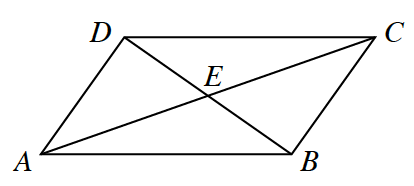### Home > CCG > Chapter 11 > Lesson 11.1.3 > Problem11-48

11-48.In the diagram at right, assume that $m∠ECB = m∠EAD$ and point $E$ is the midpoint of $\overline { A C }$. Prove that $\overline { A D }≅\overline{CB}$.

If you can prove that $\Delta DEA \cong \Delta CEB$ then you can prove $\overline{AD} \cong \overline{CB}$.

You can prove that $ΔDEA ≅ ΔCEB$ by $\text{AAS} ≅$.

Flowchart, 6 bubbles, Bubbles 1, 2, 3 on top row, 4, & 5 on middle row, and 6, on bottom row, with arrows as follows: from 1 to 4. From 2 to 4, from 3 to 5, from 5 to 4, from 4 to 6.

 Bubble 1$\enclose{circle}{ \; \\ \quad m \angle ECB = m \angle EAD \quad \\}$ Bubble 2$\enclose{circle}{ \; \\ \quad m \angle AED = m \angle CEB \quad \\}$ Bubble 3$\enclose{circle}{ \; \\ \; \\ \quad E \; \text{is the midpoint} \quad \\ \qquad \quad \text{of} \; \overline{AC}\\}$ $\text{Given} \; \Huge \searrow$ ${\text{Vertical} \; \\ \text{angles are} \; \\ \text{congruent}}\; \Huge \downarrow$ ${\Huge \downarrow} \; \text{Given}$ Bubble 4$\enclose{circle}{ \; \\ \quad \Delta AED \; \cong \; \Delta CEB \quad \\}$ $\Huge \leftarrow$ Bubble 5$\begin{array}{c} \enclose{circle}{ \; \\ \quad \overline{AE} \; \cong \; \overline{CE} \quad \\} \\ \text{Def. of midpoint} \end{array}$ ${\Huge \downarrow} \; \text{ASA}\cong$ Bubble 6$\begin{array}{l} \enclose{circle}{ \; \\ \quad \overline{AD} \; \cong \; \overline{CB} \quad \\} \\ \qquad \qquad \quad \cong \Delta \text{s} \; \rightarrow \; \; \cong \text{parts} \end{array}$